﻿ Numerical Analysis on MHD Natural Convection within Trapezoidal Cavity Having Circular Block

### Numerical Analysis on MHD Natural Convection within Trapezoidal Cavity Having Circular Block

American Journal of Applied Mathematics and Statistics

## Numerical Analysis on MHD Natural Convection within Trapezoidal Cavity Having Circular Block

Muhammad Sajjad Hossain1,, M. A. Alim2, Kazi H Kabir3

1Department of Arts and Sciences, Ahsanullah University of Science & Technology (AUST), Dhaka-1208, Bangladesh

2Department of Mathematics, Bangladesh University of Engineering & Technology (BUET), Dhaka-1000, Bangladesh

### Abstract

In this paper, we have studied MHD natural convection within trapezoidal cavity having circular block with uniformly heated bottom wall with inclination angles (ф). To investigate the effects of uniform heating with the circular block a Galerkin finite element method is studied and also used for solving the Navier-Stokes equations for different angles Φs. Here left and right walls are considered as cold and upper wall is considered as thermal insulated in a trapezoidal cavities. Rayleigh number (Ra) from 103 to 105, Hartmann number (Ha = 20) and Prandtl number (Pr) from 0.026 to 0.7 with various tilt angles Ф = 450, 300 and 00 (square) are concerned with the fluid. By different sets of governing equations along with the corresponding boundary conditions are used to set the physical problems. Results are shown in terms of streamlines, isotherms, heat flux and heat transfer rates for different Ra and Pr. It is seen that for different angles Φs conduction dominant region changes for different Pr when Ra increases. Local and average nusselt numbers are also used for heat transfer analysis for different irrespective Φs.

• Muhammad Sajjad Hossain, M. A. Alim, Kazi H Kabir. Numerical Analysis on MHD Natural Convection within Trapezoidal Cavity Having Circular Block. American Journal of Applied Mathematics and Statistics. Vol. 4, No. 5, 2016, pp 161-168. http://pubs.sciepub.com/ajams/4/5/4
• Hossain, Muhammad Sajjad, M. A. Alim, and Kazi H Kabir. "Numerical Analysis on MHD Natural Convection within Trapezoidal Cavity Having Circular Block." American Journal of Applied Mathematics and Statistics 4.5 (2016): 161-168.
• Hossain, M. S. , Alim, M. A. , & Kabir, K. H. (2016). Numerical Analysis on MHD Natural Convection within Trapezoidal Cavity Having Circular Block. American Journal of Applied Mathematics and Statistics, 4(5), 161-168.
• Hossain, Muhammad Sajjad, M. A. Alim, and Kazi H Kabir. "Numerical Analysis on MHD Natural Convection within Trapezoidal Cavity Having Circular Block." American Journal of Applied Mathematics and Statistics 4, no. 5 (2016): 161-168.

 Import into BibTeX Import into EndNote Import into RefMan Import into RefWorks

12
Prev Next

### 1. Introduction

When the heated fluid is caused to move away from the source of heat, carrying energy with it then heat transfer occurs by mass motion of a fluid. This is called convection. During the last few years, many researchers have been attracted by the well-known buoyancy driven Phenomena of convection motion of fluid. It is seen that analysis of convection such as natural convection in enclosed cavities usually is used in the engineering fields. Besides, in chemically processed industries such as solar cooling, food processing and polymer production etc. the phenomenon of natural convection and the heat and mass transfer are recurrently present. The trend of natural convection flow occurs frequently in nature by involving coupled heat and mass transfer. In these studies, the magnetohydrodynamic phenomenon having circular block is applied. Due to its diversified applications, magnetohydrodynamics (MHD) has attracted the attention of a large number of scholars. The study on natural convection flow is important in liquid-metals, electrolytes and ionized gases due to effects of magnetic field. Besides, Magnetohydrodynamics flows have applications in meteorology, solar physics, cosmic fluid dynamics, astrophysics, geophysics and in the motion of earthes core.

Many engineering applications based on natural convection in enclosed cavities [1-5] have been received crucial attention. Various initiatives have been taken to acquire a basic understanding of natural convection flows and heat transfer characteristics in a cavities reported by Patterson and Imberger , Hall et al. , Hyun and Lee , and Al-Amiri et al. . The middle-of-the-road studies dealing with convection in cavities are focused on the cases of simple geometry e.g., rectangular, square, cylindrical and spherical cavities. But the configurations of actual containers used in real life are not always simple. A few studies have been carried out on natural convection on triangular cavities filled with a viscous fluid or a porous medium by earlier researchers [10, 11, 12]. A good number of the cavities commonly used in industries are cylindrical, rectangular, trapezoidal, triangular etc. Considerable attentions have also been received by trapezoidal cavities for their applications in various fields.

A broad understanding of energy flow and entropy generation is required for an optimal process design via reducing irreversibility in terms of ‘entropy generation’. In this study, analyses on entropy generation during natural convection in a trapezoidal cavity with various inclination angles (φ = 45°, 60° and 90°) have been carried out for an efficient thermal processing of various fluids of industrial importance (Pr = 0.015, 0.7 and 1000) in the range of Rayleigh number (103 − 105) by Basak et al. . Basak et al.  studied a comprehensive heatline based approach for natural convection flows in trapezoidal enclosures accompanying the effect of various walls heating. The present numerical study focuses on natural convection flow in closed trapezoidal cavities. The energy distribution and thermal mixing in steady laminar natural convective flow through the rhombic cavities with various inclination angles, φ for various industrial applicationshas been studied by Anandalakshmi and Basak . Here simulations are carried out for various regimes of Prandtl (Pr) and Rayleigh (Ra) numbers. Dimensionless streamfunctions and heatfunctions have been used to visualize the flow and energy distribution, respectively. Hasanuzzaman et al.  studied a computational numerical work to see the effects of magnetic field on natural convection for a trapezoidal cavity. To investigate the effects, finite element method is used to solve the governing equations for different parameters such as Rayleigh number, Hartmann number and inclination angle of inclined wall of the cavity using both inclined walls and bottom wall have constant temperature where the bottom wall temperature is higher than the inclined walls and top wall of the cavity is adiabatic. It is found that heat transfer decreased as φ increases from 0 to 60 and also Ha increases from 0 to 50 at Ra = 105 and 106 respectively.

Selimefendigil et al.  investigated entropy generation due to natural convection in entrapped trapezoidal cavities filled with nanofluid under the influence of magnetic field where the upper (lower) cavity is filled with CuO-water (Al2O3-water) nanofluid and the top and bottom horizontal walls of the trapezoidal cavities are maintained at constant hot temperature while other inclined walls of the enclosures are at constant cold temperature and also different combinations of Hartmann numbers are imposed on the upper and lower trapezoidal cavities. It is found that the average heat transfer reduction with magnetic field is more pronounced at the highest value of the Rayleigh number when there is no magnetic field in the lower cavity. Besides, the average Nusselt number enhances as the value of the Hartmann number of the upper cavity increases. It is also found that the heat transfer enhancement rates with nanofluids which are in the range of 10% and 12% are not affected by the presence of the magnetic field.

Gireesha et al.  performed the geometry of an unsteady viscous incompressible fluid with uniform distribution of dust particles through a long rectangular channel under the influence of time-dependent periodic pressure gradient. Initially the fluid and dust particles are at rest. Analytical expressions for velocities of fluid and dust particles are obtained by solving the partial differential equations using variable separation method.

At the same time, Hossain and Alim [19, 20] have studied MHD free convection within trapezoidal cavity with uniformly and non-uniformly heated bottom wall. They have analyzed free convection within a trapezoidal cavity for nonuniformly and uniformly heated bottom wall, insulated top wall and isothermal side walls with inclination angles (ф). The fluid is concerned for the wide range of Rayleigh number (Ra) from 103 to 107and Prandtl number (Pr) from 0.026-1000 with various tilt angles Ф = 450, 300 and 00 (square). The properties of the fluid were presumed to be constant. The physical problems are presented mathematically by different sets of governing equations along with the corresponding boundary conditions. The non-dimensional governing equations are discretized by using Galerkin weighted residual method of finite element formulation. Results are presented in terms of streamlines, isotherms, and average and Local Nusselt numbers, for different parameters namely Prandtl number Pr and Rayleigh number Ra. This range of Ra is selected on the basis of calculation covering natural convection dominated regimes. The results indicate that the Local and average Nusselt number at the uniform heating of bottom wall of the cavity depend on the dimensionless parameters. Also Hossain et al.  have performed finite element analysis of MHD Free Convection within trapezoidal enclosures with uniformly heated side walls.

Natarajan et al.  have studied the combined natural convection and surface radiation heat transfer in a solar trapezoidal cavity absorber for Compact Linear Fresnel Reflector (CLFR). In this study the numerical simulation results are presented in terms of Nusselt number correlation to show the effect of these parameters on combined natural convection and surface radiation heat loss. The natural convection in a porous trapezoidal enclosure for uniformly or non-uniformly heated bottom wall is also examined by Basak et al. . In this study, penalty finite element analysis with bi-quadratic elements is used for solving the Navier–Stokes and energy balance equations. The numerical solutions are studied in terms of streamlines, isotherms, heatlines, local and average Nusselt numbers for a wide range of parameters Da(10−5–10−3), Pr(0.015–1000) and Ra(Ra = 103–106). At low Darcy number (Da = 10−5), heat transfer is primarily due to conduction for all φ’s as seen from the heatlines which are normal to the isotherms.

Basak et al.  have also examined heat flow patterns in the presence of natural convection within trapezoidal cavities with heatlines concept. In this lesson, natural convection within a trapezoidal cavity for uniformly and non-uniformly heated bottom wall, insulated top wall and isothermal side walls with inclination angle has been investigated. Momentum and energy transfer are characterized by stream functions and heat functions, respectively, whereas, stream functions and heat functions satisfy the dimensionless forms of momentum and energy balance equations, respectively. Finite element method has been used to solve the velocity and thermal fields and the method has also been proved robust to obtain the stream function and heat function accurately. The unique solution of heatfunctions for situations in differential heating is a strong function of Dirichlet boundary condition which has been obtained from average Nusselt numbers for hot or cold regimes.

Trapezidal cavities having circular block have not been used in the literature yet. In this study, heat flow analysis for MHD natural convection using circular block with uniformly heated bottom wall has been studied for trapezoidal cavities. Results are shown to display the circulations and for different physical parameters Ra, Pr and Ha in terms of streamlines, stream functions, total heat flux, isotherms and heat transfer rates for the walls in terms of average and local nusselt numbers.

### 2. Physical Model

The Physical model is well thought-out of height L with the left wall inclined at an angle ф = 450, 300, 00 with Y axis in a two-dimensional trapezoidal cavity having circular block which is schametically shown in Figure 1. Here left wall and right (side) walls are subjected to cold Tc temperature; bottom wall is subjected to hot Th temperature while the top wall is kept insulated. The boundary conditions for velocity are considered as no-slip on solid boundaries.Download asVeiw figureFigures index
Figure 1. Schematic diagram of the physical system for (a) ф = 450 (b) ф = 300 and (c) ф = 00
2.1. Mathematical Formulation

The flow inside the cavity is assumed to be two-dimensional, steady, laminar and incompressible and the fluid properties are said to be constant. The momentum equation, Boussinesq approximation is used for the treatment of buoyancy term.The dimensionless governing equations describing the flow are as follows:(1)(2)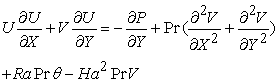(3)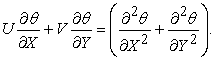(4)

To write equations (1-4), the following definitions and dimensionless parameters are used,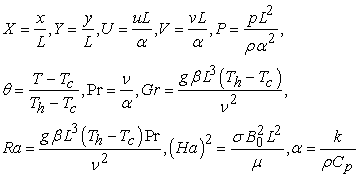(5)
2.2. Boundary Conditions

The boundary conditions  (also shown in Figure 1), for the present problem are specified as follows:

At the bottom wall: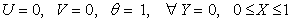(6)

At the left wall: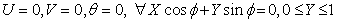(7)

At the right wall:(8)

At the top wall: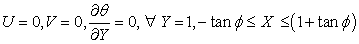(9)

where X and Y are dimensionless coordinates varying along horizontal and vertical directions, respectively; U and V are dimensionless velocity components in X and Y directions, respectively;is the dimensionless temperature.

Circular block is also used inside the trapezoidal cavity.

The local Nusselt number at the heated surface of the cavity which is defined by the following expression:where n denotes the normal direction on a plane. The non-dimensional stream function is defined as,The average Nusselt number at the cold left and right (side) walls, uniformly heated bottom wall and insulated top walls of the cavities based on the non-dimensional variables may be expressed as,2.3. Numerical Methodology

To solve differential equations numerically finite element analysis is a method which can be applied to many problems in engineering and scientific fields. Finite element simulation of natural convection in a two-dimensional trapezoidal cavities having circularblock has been studied here. This research starts from two-dimensional Navier-Stoke’s equations together with the energy equation to obtain the corresponding finite element equations.Galerkin’s weighted resudal method is applied to discretize the non-dimentional governing equations. Triangular mesh is used to obtain the solution. Because this type of mesh can be used in any shape of domain .Details of method are available in Taylor and Hood  and Dechaumphai .

### 3. Grid Independence Test

#### Table 1. Grid Sensitivity Check at Pr = 0.7, Phi =450, Ha = 50 and Ra = 105

To determine the proper grid size for this study, a grid independence test is conducted in the above Table 1 with Pr = 0.7, Phi =450, Ha=50 and Ra = 105. The following five types of mesh are considered for the grid independence test.These grid densites are 3597, 4840, 5718, 10618, 13954 nodes and 520, 708, 842, 1592, 2080 elements. Average Nusselt numbers at the heated surface study of trapezoidal enclosures are used as a measure of accuracy of solution. From the Table 1, a grid size of 10614 nodes and 1592 elements is chosen for better accuracy.

### 4. Code Validation

#### Table 2. Code validation for uniform heating of side wall with Pr = 0.7

To compare the current code results, the present numerical solution is validated against the numerical result of Basak et al.  for natural convection in a trapezoidal cavity for streamlines, isotherms and heatflux. For three different Rayleigh numbers (Ra = 103, 104 and 105), while the prandtl number and angle are fixed i.e. Pr = 0.7, ф = 450 for uniform heating of side wall, average Nusselt number is calculated for 1566 nodes and 222 elements. The numerical solutions (present work and Basak et al. ) are in good agreement, which is shown in Table 2.

### 5. Results and Discussion

Numerical results for different Rayleigh number Ra = 103- 105 and Prandtl number, Pr = 0.026, 0.7 and Hartmann number Ha=50 for streamlines, isotherms and heat function or heatflux for the fluid with various angles, ф = 450, 300, 00 is presented here for MHD natural convection with trapezoidal cavity having circular block. These are shown in Figure 2 - Figure 4. Also, heat transfer rate for local and average nusselt numbers are shown for various values of Rayleigh and Prandtl numbers and angles ф.

5.1. Uniform Heating

Figure 2 shows the effects of streamlines, isotherms and heat function or total heat flux for Rayleigh numbers. It is noted that stream function’s magnitude varying from smaller to bigger which occur due to heated and for the low Rayleigh number, heat flow is occurred due to conduction. Various vortices are shown in streamlines due to heat conduction. Isotherms are seen likely smooth curves and symmetric with respect to vertical symmetrical line and take place symmetrically along side (left or right) walls for Ra = 103, Pr = 0.026 and ф = 00(square cavity) (Figure 2a). Again, temperature contours come about symmetrically near the side walls of the enclosure and curves are smooth and symmetric with respect to central symmetrical line for Ra = 103, Pr = 0.026 and ф = 300 (Figure 2b). Also for Ra = 103, Pr = 0.026 and ф = 450 isotherms (temperature) arise symmetrically near the side walls of the cavity and also curves are smooth and symmetric with respect to vertical symmetrical line (Figure 2c). Temperature distribution for various ф in trapezoidal cavity for circular block has been exhibited by the pressure of significant convection. Heat flux or heat function governed the distribution of heat energy that observed for uniform heating cases. Besides, various types of vortices are seen in panels of Figure 2a-c. The heatlines or total heat flux or heat functions are also shown in panels of Figure 2a-c. The heatlines illustrate similar attribute that were also observed for uniform heating cases.

The fascinating impact is that ф = 00(square cavity) is finer to ф = 450 and 300 at the bottom corner point. Besides, near the bottom portion of side walls heatlines are more dense for ф = 450 and less dense for ф = 00(square cavity). The rate of heat transfer from the bottom to the side walls are being enhanced by dense heatlines. It is noted that from bottom edges to central symmetrical line the magnitudes of heat function or heat flux decreases at the top portion of the cavity for ф = 450 and 300 heat transfer is higher condensed to ф = 00(square cavity) based on value of heatfunction (Π). The heat transfer is quite large at the corners of bottom wall, the thermal boundary layer is found to develop near the bottom edges and at the top portion of the cold wall boundary layer’s thickness is bigger signifying less heat transfer to the top portion.

Figure 3 shows that streamfunction’s the magnitude are smaller for Ra = 105 and Pr = 0.026 and isotherms occur symmetrically near the side walls of the cavity and curves are also smooth and symmetric with respect to central symmetrical line for Ra = 105, Pr = 0.026 and ф = 450, 300, 00(square cavity) (Figure 2a-c, Figure 3a-c) and the rate of heat transfer are being enhanced by heatlines from the bottom to side walls for Ra = 104 and Pr = 0.026. At critical Ra the middle portion of isotherms starts getting deformed and the maximum value of ψ is at the eye of vortices. If Ra is increased, the buoyancy driven circulation inside the cavity has also increased which are seen from greater magnitudes of stream function (Figure 3).Download asVeiw figureFigures index
Figure 2. Stream function (Ψ), temperature (θ), heat function or total heat flux (П) for θ(X,0) = 1 with Pr = 0.026, Ha = 50 and Ra = 103 (a) Φ = 0o (b) Φ = 30o (c) Φ = 45oDownload asVeiw figureFigures index
Figure 3. Stream function (Ψ), temperature (θ), heat function or total heat flux(П) for θ(X,0) = 1 with Pr = 0.026, Ha = 50 and Ra = 105(a) Φ = 0o (b) Φ = 30o (c) Φ = 45oDownload asVeiw figureFigures index
Figure 4. Stream function (Ψ), temperature (θ), heat function or total heat flux(П) for θ(X,0) = 1 with Pr = 0.7, Ha = 50 and Ra = 105 (a) Φ = 0o (b) Φ = 30o (c) Φ = 45o

The streamfunction’s magnitude is circular or elliptical near the core but the streamlines is almost parallel near to the side wall exhibiting large intensity of flow for Pr = 0.7 and Ra = 105 which is shown in Figure 4. Vortices are also showy for every case of cavities. Also for Pr = 0.7 and Ra = 105 isotherms are likely symmetrical near the side walls of the cavity and curves are flat symmetric with respect to central symmetrical line for ф = 450, 300, 00(square cavity). The detection is that for irrespective of angles ф the intensity of flow has been increased as seen in Figure 3. Although for intensity of flow streamlines near the wall is almost parallel to wall but streamlines look like circular or elliptical near the core (see Figure 3). It is captivating that for Pr = 0.7 and Ra = 105 multiple correlations are deficient. Isotherms are highly compressed near the side walls in order to enhanced flow circulations except near the bottom wall especially for ф = 450 and 300. The large temperature gradient near the side walls are due to significant number of heatlines with a large variation of heatfunction as seen in Figure 4a-c .

5.2. Heat Transfer Rates: Local Nusselt Numbers (Nulocal) and Average Nusselt Numbers (Nuav)Download asVeiw figureFigures index
Figure 5. Variations of local Nusselt numbers (Nulocal) with distance for different Rayleigh numbers, Ra = 103, 104, 105 and angles (a) Φ = 0o, (b) 45o when Pr = 0.7 for uniform heating of bottom wall

Figure 5(a,b) shows the effect of local heat transfer rates i.e. Nulocal vs distance for various inclination tilt angles i.e. for ф = 00, 450 when Ra = 103, 104, 105 and Pr = 0.7 having circular block for uniform heating of bottom wall. Local heat transfer rate is maximum near the edge of side wall and minimum near to the bottom edge of the wall due to the heated bottom wall, cold side walls and insulated top wall. It is seen that for (a) ф = 00 Nulocal heat transfer rates are slightly same near to the side wall but slightly different near to the edge of the bottom wall and the boundary layer starts to form at the bottom edge of the side wall . In order to large intensity of convection, (b) ф = 450 at Ra = 103, 104, 105 there is more change of heat transfer rates near to the side wall and also near to the bottom edge of the wall. As Ra increases, magnitudes of local heat transfer rates become smaller and maximum heat transfer occurs from one edge of the side wall continuing bottom wall to the other edse of the side wall. It may be mentioned that the larger degree of compression of isotherms for uniform heating case results in larger and Nulocal is quite large near to the bottom wall.

The heat transfer rates are illustrated for uniform heating of bottom wall having circular block in Figure 6(a,b), where distributions of average Nusselt number are plotted vs the logarithmic Rayleigh number respectively. It may be noted that average Nusselt number is obtained by considering temperature gradient. It may also be noted that as Ra increases then the average Nusselt number increases. It is seen in Figure 6(a) that as Ra increases from 103-105 then average Nusselt number is straightly moving for Φ = 0o , 300 but for Φ = 45o Ra increases more when Pr = 0.026. As Pr increases (Figure 6(b)) then conduction dominant heat transfer is narrowed down.

It is also seen from Figure 6(b) that, as Pr increases more then from uniform heating case it is analyzed that average Nusselt number for bottom wall is also slightly increasing during the entire Rayleigh number regime.. As Pr increases then for conduction dominant heat transfer, the average Nusselt number is generally constant irrespective of Ra. It is observed that Nuav at the middle portion of bottom wall for Φ = 30o is slightly changing for uniform heating case whereas for Φ = 30o and 45o heat transfer rates are lightly identical.Download asVeiw figureFigures index
Figure 6. Variations of Average Nusselt Number vs Rayleigh number for (a) Pr = 0.026, (b) Pr = 0.7 and angles Φ = 0o, 30o, 45o for uniform heating of bottom wall

### 6. Conclusion

In this revise, the predicament of MHD natural convection having circular block within trapezoidal cavities for uniformly heated bottom walll with heatlines concept has been prsented numerically in terms of streamlines and isotherms and heat function or total heat flux with the aid of flow and temperature field for different parameters Pr, Ra and Ha. The results of the numerical analysis lead to the following conclusions:

• Due to the circular block, at low Rayleigh number (Ra), the circulation outline into the trapezoidal cavity is very fragile because the buoyancy forces are being dominated over the viscous forces. Finally a clear mystification occurs in the contour of re-circulating vortices as Rayleigh number (Ra) increases since the buoyancy forces are dominating over the viscous forces.

• When the Rayleigh number (Ra) increases then due to Ha and circular block of the cavity, a little bit anomalies of the isothermlines increases.

• Near the edge of the wall, the local heat transfer rate is maximum but minimum near the center of the wall irrespective of all angles (ф) for uniform heating of the bottom wall for Rayleigh number (Ra) 103 to 105 gradually.

• The heat transfer rate average Nusselt Number, Nuav increases with the increase of Rayleigh number, Ra, for uniform heating of bottom wall.

• The maximum rate of heat transfer is obtained for the highest value Ra and also Pr and heat transfer rate depends on also Pr.

### Acknowledgements

Authors would like to express their gratitude to the Department of Mathematics, Bangladesh University of Engineering and Technology (BUET) and Department of Arts and Sciences, Ahsanullah University of Science and Technology (AUST), Dhaka, Bangladesh, for providing computing facility during this work.

### Nomenclature

B0: Magnetic induction

Cp: Specific heat at constant pressure (J/kg K)

G: Gravitational acceleration (m/s2)

Gr: Grashof number

H: Convective heat transfer coefficient (W/m2 K)

Ha: Hartmann number

K: Thermal conductivity of fluid(W/m K)

L: Height or base of trapezoidal cavity (m)

k: Thermal conductivity ratio fluid

N: Total number of nodes

Nuav: : Average Nusselt number

Nulocal: Local Nusselt number

P: Non-dimensional pressure

p: Pressure

Pr: Prandtl number

Ra: Rayleigh number

T: Non-dimensional temperature

Th: Temperature of uniformly heated bottom wall (K)

Tc: Temperature of cold vertical wall (K)

U: x component of dimensionless velocity

u: x component of velocity (m/s)

V: y component of dimensionless velocity

v: y component of velocity (m/s)

V0: Lid Velocity

x, y: Cartesian Coordinates

X, Y: Dimensionless Cartesian coordinates

Greek symbols

α: Thermal diffusivity (m2/s)

β: Coefficient of thermal expansion (K-1)

ρ: Density of the fluid (kg/m3)

∆θ: Temperature difference

Θ: Fluid temperature

μ: Dynamic viscosity of the fluid (Pa s)

Π: Heatfunction

ν: Kinematic viscosity of the fluid (m2/s)

σ: Fluid electrical conductivity(Ω-1m-1)

Subscripts

b: Bottom wall

l: Left wall

r: Right wall

s: Side wall

### References

  J.M. Garrpeters, The neutral stability of surface-tension driven cavity flows subject to buoyant forces-I. Transverse and longitudinal disturbances, Chem. Eng. Sci. 47 (5), 1992, 1247-1264.In article View Article  L.B. Wang, N.I. Wakayama, Control of natural convection in non- and low-conducting diamagnetic fluids in a cubical enclosure using inhomogeneous magnetic fields with different directions, Chem. Eng. Sci. 57 (11), 2002, 1867-1876.In article View Article  I.E. Sarris, I. Lekakis, N.S. Vlachos, Natural convection in a 2D enclosure with sinusoidal upper wall temperature, Num Heat Transfer A 42 (2002) 513-530.In article View Article  A. Ousegui, A. Le Bail, M. Havet, Numerical and experimental study of a natural convection thawing process, AIChE J. 52 (12), 2006, 4240-4247.In article View Article  O.G. Martynenko, P.P. Khramtsov, Free-Convective Heat Transfer, Springer, Berlin, 2005.In article PubMed  J. Patterson, J. Imberger, Unsteady natural convection in a rectangular cavity, J.Fluid Mech., 100, 1980, 65-86.In article View Article  J.D. Hall, A. Bejan, J.B. Chaddock, Transient natural convection in a rectangular enclosure with one heated side wall, Int. J. Heat Fluid Flow, 9, 1988, 396-404.In article View Article  J.M. Hyun, J.W. Lee, Numerical solutions of transient natural convection in a square cavity with different sidewall temperature, Int. J. Heat Fluid Flow, 10, 1989, 146-151.In article View Article  A.M. Al-Amiri, K.M. Khanafer, Pop I, Numerical simulation of combined thermal and mass transport in a square lid-driven cavity, Int. J. Thermal Sci., 46(7), 2007, 662-671.In article View Article  Y.E. Karyakin, Y.A. Sokovishin, O.G. Martynenko, Transient natural convection in triangular enclosures, Int. J. Heat Mass Transfer, 31, 1988, 1759-1766.In article View Article  A. Bejan, D. Poulikakos, Natural convection in an attic shaped space filled with porous material, J. Heat Transfer – Trans. ASME, 104, 1982, 241-247.In article  D. Poulikakos, A. Bejan, Numerical study of transient high Rayleigh number convection in an attic-shaped porous layer, J. Heat Transfer – Trans. ASME, 105, 1983, 476-484.In article  T. Basak, R. Anandalakshmi, Kumar Pushpendra, S. Roy, “Entropy generation vs energy flow due to natural convection in a trapezoidal cavity with isothermal and non-isothermal hot bottom wall”, Energy, 37(1), January 2012, 514.In article View Article  T. Basak, D. Ramakrishna, S. Roy, A. Matta, I. Pop, “A comprehensive heatline based approach for natural convection flows in trapezoidal enclosures: Effect of various walls heating”, International Journal of Thermal Sciences, 50 (8), August 2011, 1385-1404.In article View Article  R. Anandalakshmi, T. Basak, “Heat flow visualization for natural convection in rhombic enclosures due to isothermal and non-isothermal heating at the bottom wall”, International Journal of Heat and Mass Transfer, 55(4), 31 January 2012, 1325-1342.In article View Article  M. Hasanuzzaman, H.F. Öztop, M. M. Rahman, N.A. Rahim, R. Saidur and Y. Varol,“Magnetohydrodynamic natural convection in trapezoidal cavities”,  International Communications in Heat and Mass Transfer, 39(9), November 2012, 1384-1394.In article View Article  F. Selimefendigil, H. F. Öztop and N. Abu-Hamdeh “Natural convection and entropy generation in nanofluid filled entrapped trapezoidal cavities under the influence of magnetic field”, Entropy 2016, 18(2), 43.In article View Article  B. J. Gireesha, C.S. Bagewadi, B.C. Prasannakumar, “Study of unsteady dusty fluid Flow through rectangular channel in Frenet Frame Field system”, International Journal of Pure And Applied Mathematics, 34 (4), January 2007, 525-535.In article  M. S. Hossain, M. A. Alim, “MHD free convection within trapezoidal cavity with non-uniformly heated bottom wall”, International Journal of Heat and Mass Transfer, 69, February 2014, 327-336.In article View Article  M. S. Hossain, M. A. Alim, “MHD free convection within trapezoidal cavity with uniformly heated bottom wall”, Annals of Pure and Applied Mathematics, 3(1), 2013, 41-55.In article  M. S. Hossain, M. N. Uddin, M. A. Alim, M. Shobahani, “Finite Element Analysis of MHD Free Convection within trapezoidal Eenclosures with uniformly heated side walls.”, IOSR Journal of Mathematics, 6(3), May-Jun 2013, 64-73.In article View Article  S. K. Natarajan, K.S. Reddy, and T. K. Mallick, “Heat loss characteristics of trapezoidal cavity receiver for solar linear concentrating system”, Applied Energy, 93, May 2012, 523-531.In article View Article  T. Basak, S. Roy, A. Matta and I. Pop, “Analysis of heatlines for natural convection within porous trapezoidal enclosures: Effect of uniform and non-uniform heating of bottom wall” International Journal of Heat and Mass Transfer, Vol. 53, Issues 25-26, December 2010, 5947-5961.In article View Article  T. Basak, S. Roy, and I. Pop, Heat flow analysis for natural convection within trapezoidal enclosures based on heatline concept, Int.J. Heat Mass Transfer, 52, March 2009, 2471-2483.In article View Article  C. Taylor and P. Hood, “A Numerical Solution of the Navier-Stokes Equations Using Finite Element echnique”, Computer and Fluids, 1( 1), 1973, 73-100.In article View Article  P. Dechaumphai, Finite Element Method in Engineering, 2nd ed., Chulalongkorn University Press, Bangkok, 1999.In article